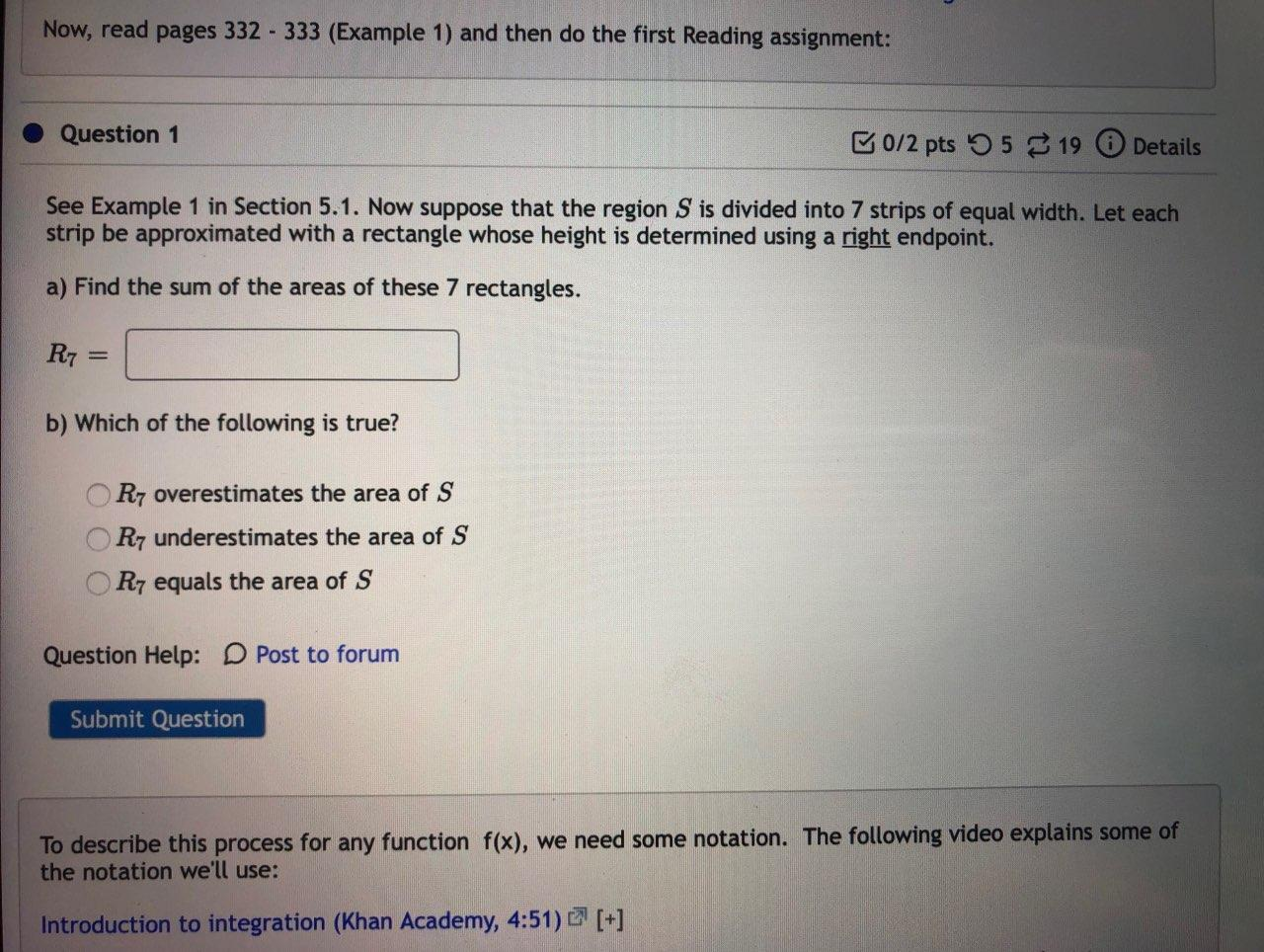### Create an Account

Home / Questions / Now, read pages 332 - 333 (Example 1) and then do the first Reading assignment: Question 1...

# Now, read pages 332 - 333 (Example 1) and then do the first Reading assignment: Question 1 G0/2 pts 55 19 Details See Example 1 in Section 5.1. Now suppose that the region S is divided into 7 strips o

Now, read pages 332 - 333 (Example 1) and then do the first Reading assignment: Question 1 G0/2 pts 55 19 Details See Example 1 in Section 5.1. Now suppose that the region S is divided into 7 strips of equal width. Let each strip be approximated with a rectangle whose height is determined using a right endpoint. a) Find the sum of the areas of these 7 rectangles. R7 b) Which of the following is true? R7 overestimates the area of S R7 underestimates the area of S R7 equals the area of S Question Help: D Post to forum Submit Question To describe this process for any function f(x), we need some notation. The following video explains some of the notation we'll use: Introduction to integration (Khan Academy, 4:51) [+]See Example 1 in Section 5.1. Now suppose that the region SS is divided into 7 strips of equal width. Let each strip be approximated with a rectangle whose height is determined using a right endpoint.
(Use rectangles to estimate the area under the parabola y= x^2 from 0 to 1)
a) Find the sum of the areas of these 7 rectangles.

R7=

b) Which of the following is true?

• R7R7 overestimates the area of SS
• R7R7 underestimates the area of SS
• R7R7 equals the area of SS

Jun 04 2021 View more View LessSubscribe To Get Solution